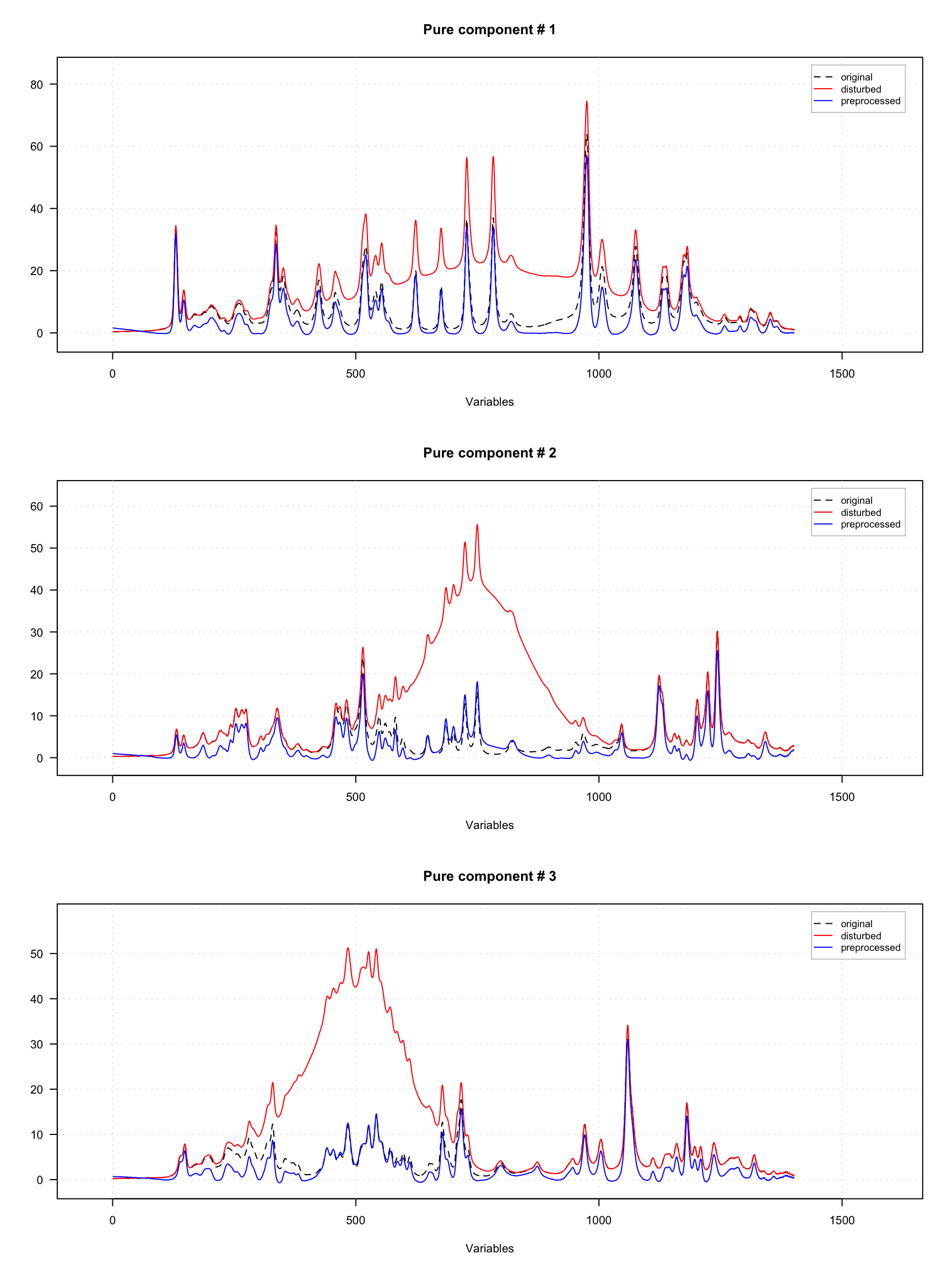## Variable selection as preprocessing method

Variable selection can be done by using mda.exclcols(), which simply hides variables/columns, which must not be taken into account in calculations, or by mda.subset() which selects only desired columns and remove the rest. Both methods preserve all additional attributes assigned to the data.

The method prep.varsel() is simply a wrapper, which allows selection of only desired variables (similar to mda.subset()) but can be also incorporated into preprocessing workflow (see next section for details). In the example below it is used to select only even columns from the data matrix.

# load spectra from the Simdata and add some attributed
data(simdata)
X <- simdata$spectra.c attr(X, "xaxis.values") <- simdata$wavelength
attr(X, "xaxis.name") <- "Wavelength, nm"
attr(X, "name") <- "Simdata"

# apply variable selection as preprocessing
Y <- prep.varsel(X, seq(2, ncol(X), by = 2))

# show both original and preprocessed spectra
par(mfrow = c(2, 1))
mdaplot(X, type = "l")
mdaplot(Y, type = "l")You can notice that on the second plot the lines are not smooth anymore as the number of points is twice smaller.